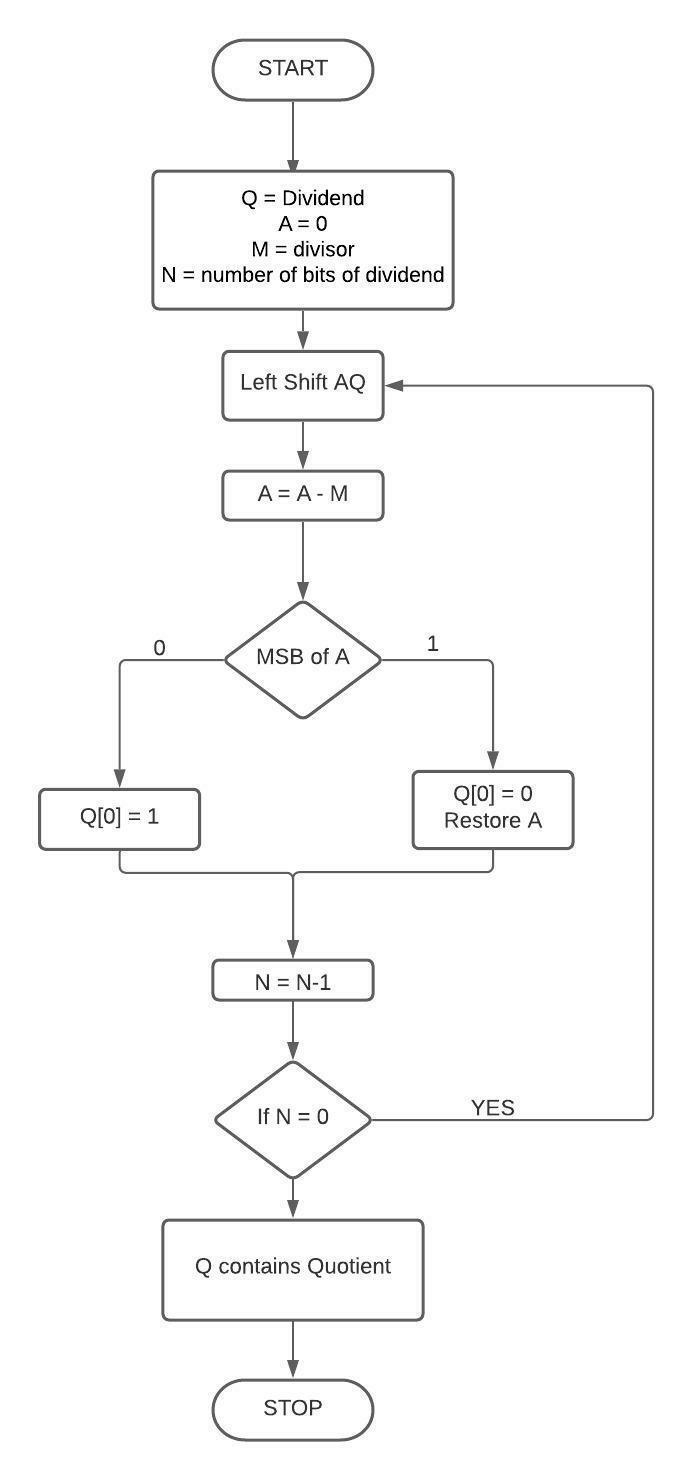# Restoring Division Algorithm For Unsigned Integer in C++

C++Server Side ProgrammingProgramming

Discuss dividing an unsigned integer using a division algorithm. Some division algorithms are applied on paper, and others are implemented on digital circuits. Division algorithms are of two types: slow division algorithm and fast division algorithm. Slow division algorithm includes restoring, non-performing restoring, SRT, and non-restoring algorithm.

In this tutorial, we will discuss the Restoring algorithm, assuming that 0 < divisor < dividend.

## Approach to Find the Solution

In this, we will use register Q to store quotient, register A to store remainder, and M to store divisor. The initial value of A is kept at 0, and its value is restored, which is why this method is restoring division.

• Initialize registers with values,

• Q = Dividend,

• A = 0,

• M = divisor,

• N = number of bits of dividend.

• Left shift AQ means taking register A and Q as a single unit.

• Subtract A with M and store in A.

• Check the most significant bit of A:

• If it is 0, set the least significant bit to 1.

• Else, set the least significant bit to 0.

• Restore the value of A and decrement the value of counter N.

• If N = 0, break the loop; otherwise, go to step 2.

• The quotient is stored in register Q.

## Flow Chart## Example

C++ Code for the Above Approach

#include <iostream>
using namespace std;
int main(){
// initializing all the variables with Dividend = 9, Divisor = 2.
int Q = 8,q=1,M=3;
short N = 4;
int A = Q;
M <<= N;
// loop for division by bit operation.
for(int i=N-1; i>=0; i--) {
A = (A << 1)- M;
// checking MSB of A.
if(A < 0) {
q &= ~(1 << i);  // set i-th bit to 0
A = A + M;
} else {
q |= 1 << i;     // set i-th bit to 1
}
}
cout << "Quotient: "<< q;
return 0;
}

## Output

Quotient: 2

## Conclusion

In this tutorial, we discussed the Restoring division algorithm for an unsigned integer. We discussed a simple approach to solve this problem with the help of a flow chart and applying bit operations. We also discussed the C++ program for this problem which we can do with programming languages like C, Java, Python, etc. We hope you find this tutorial helpful.